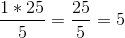# PSAT Math : How to find fractional percentages

## Example Questions

### Example Question #1 : How To Find Fractional Percentages

You have 2 fair dice that each have 6 faces. On one roll, what's the probability that both dice land on an even number?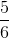Explanation:

In order to find the probability of rolling two even numbers on 2 dice we need to find the probability of each dice having an even number on the roll and then multiply them together.

The probability of rolling an even number is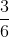because 2, 4, and 6 are even numbers which goes in the numerator and there are 6 total numbers which goes in the denominator. After this we reduce the fraction and get one half. Then we need to muliply this by probability of the second dice having an even number.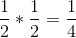### Example Question #1 : How To Find Fractional Percentages

What is 15% of a \$16.73 bill?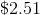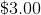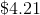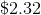Explanation:

We can re write 15% as a fraction and then use proportions to solve.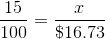From here we cross multiply and divide to solve for.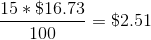### Example Question #6 : Fractions And Percentage

If a test has a total ofquestions andof the questions are multiple choice, how many questions are multiple choice?Explanation:

To solve this problem we set up a ratio. We want to findof. Therefore, we set up the following ratio: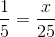In the caserepresents the number of questions that are multiple choice. From here we cross multiply and divide.